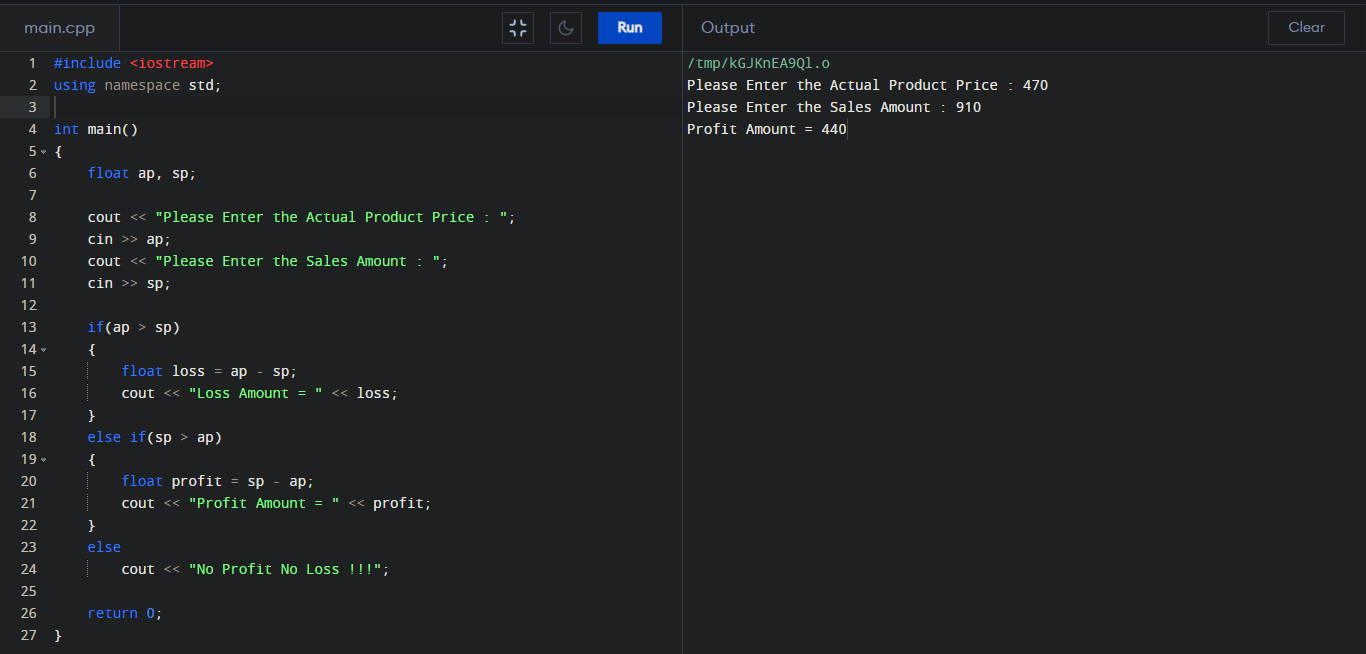C++ Program to Calculate Profit or Loss

How can we get the Profit or Loss amount? We can get the Profit or Loss amount by subtracting Sell price from Actual price.

Profit or Loss calculation using C++ :

#include <iostream>
using namespace std;

int main()
{
float ap, sp;

cout << "Please Enter the Actual Product Price : ";
cin >> ap;
cout << "Please Enter the Sales Amount : ";
cin >> sp;

if(ap > sp)
{
float loss = ap - sp;
cout << "Loss Amount = " << loss;
}
else if(sp > ap)
{
float profit = sp - ap;
cout << "Profit Amount = " << profit;
}
else
cout << "No Profit No Loss !!!";

return 0;
}

Output :